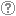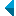# Search the repositoryQuery: search in TitleAuthorAbstractKeywordsFull textYear of publishing ANDORAND NOT search in TitleAuthorAbstractKeywordsFull textYear of publishing ANDORAND NOT search in TitleAuthorAbstractKeywordsFull textYear of publishing ANDORAND NOT search in TitleAuthorAbstractKeywordsFull textYear of publishing Work type: All work types Habilitation (m4) Specialist thesis (m3) High school thesis (m6) Bachelor work * (dip) Master disertations * (mag) Doctorate disertations * (dok) Research Data or Corpuses (data) * old and bologna study programme Language: All languagesSlovenianEnglishGermanCroatianSerbianBosnianBulgarianCzechFinnishFrenchGerman (Austria)HungarianItalianJapaneseLithuanianNorwegianPolishRussianSerbian (cyrillic)SlovakSpanishSwedishTurkishUnknown Search in: RUP    FAMNIT - Faculty of Mathematics, Science and Information Technologies    FHŠ - Faculty of Humanities    FM - Faculty of Management    FTŠ Turistica - Turistica – College of Tourism Portorož    FVZ - Faculty of Health Sciences    IAM - Andrej Marušič Institute    PEF - Faculty of Education    UPR - University of Primorska    ZUP - University of Primorska PressCOBISS    Fakulteta za humanistične študije, Koper    Fakulteta za management Koper in Pedagoška fakulteta Koper    Fakulteta za vede o zdravju, Izola    Knjižnica za tehniko, medicino in naravoslovje, Koper    Turistica, Portorož    Znanstveno-raziskovalno središče Koper Options: Show only hits with full text Reset

 1 - 10 / 6812345671.Economics and mathematical theory of gamesAjda Fošner, 2012, original scientific articleAbstract: The theory of games is a branch of applied mathematics that is used in economics, management, and other social sciences. Moreover, it is used also in military science, political science, international relations, computer science, evolutionary biology, and ecology. It is a field of mathematics in which games are studied. The aim of this article is to present matrix games and the game theory. After the introduction, we will explain the methodology and give some examples. We will show applications of the game theory in economics. We will discuss about advantages and potential disadvantages that may occur in the described techniques. At the end, we will represent the results of our research and its interpretation.Found in: ključnih besedahSummary of found: Zadetek v naslovuKeywords: the theory of games, matrix games, economicsPublished: 15.10.2013; Views: 3913; Downloads: 77This document has more files! More... 2.On bipartite Q-polynomial distance-regular graphs with c [sub] 2 [equal] 1Štefko Miklavič, 2007, original scientific articleAbstract: Let ▫$\Gamma$▫ denote a bipartite ▫$Q$▫-polynomial distance-regular graph with diameter ▫$d \ge 3$▫, valency ▫$k \ge 3$▫ and intersection number ▫$c_2=1$▫. We show that ▫$\Gamma$▫ has a certain equitable partition of its vertex set which involves ▫$4d-4$▫ cells. We use this partition to show that the intersection numbers of ▫$\Gamma$▫ satisfy the following divisibility conditions: (I) ▫$c_{i+1}-1$▫ divides ▫$c_i(c_i-1)$▫ for ▫$2 \le i \le d-1$▫, and (II) ▫$b_{i-1}-1$▫ divides ▫$b_i(b_i-1)$▫ for ▫$1 \le i \le d-1$▫. Using these divisibility conditions we show that ▫$\Gamma$▫ does not exist if ▫$d=4$▫.Found in: ključnih besedahSummary of found: ...mathematics, grah theory, distance-regular graphs, ▫$Q$▫-polynomial property, equitable partitions... Keywords: mathematics, grah theory, distance-regular graphs, ▫$Q$▫-polynomial property, equitable partitionsPublished: 15.10.2013; Views: 3262; Downloads: 35Full text (0,00 KB) 3.Hamiltonicity of vertex-transitive graphs of order 4pKlavdija Kutnar, Dragan Marušič, 2008, original scientific articleAbstract: It is shown that every connected vertex-transitive graph of order ▫$4p$▫, where ▫$p$▫ is a prime, is hamiltonian with the exception of the Coxeter graph which is known to possess a Hamilton path.Found in: ključnih besedahSummary of found: ...graph theory, vertex-transitive graphs, Hamilton cycle, automorphism group... Keywords: graph theory, vertex-transitive graphs, Hamilton cycle, automorphism groupPublished: 15.10.2013; Views: 2877; Downloads: 34Full text (0,00 KB) 4.Coding theory and applications, cyclic codesEnes Pašalić, 2013, other educational materialFound in: ključnih besedahSummary of found: Zadetek v naslovuKeywords: coding theory, cyclic codes, BCH codes, Reed-Solomon codes, channel erasuresPublished: 15.10.2013; Views: 2367; Downloads: 103Full text (0,00 KB)This document has more files! More... 5.Isomorphism checking of I-graphsTomaž Pisanski, Boris Horvat, Arjana Žitnik, 2012, original scientific articleAbstract: We consider the class of ▫$I$▫-graphs, which is a generalization of the class of the generalized Petersen graphs. We show that two ▫$I$▫-graphs ▫$I(n, j, k)$▫ and ▫$I(n, j_1, k_1)$▫ are isomorphic if and only if there exists an integer ▫$a$▫ relatively prime to $n$ such that either ▫$\{j_1, k_1\} = \{aj \mod n, \; ak \mod n \}$▫ or ▫$\{j_1, k_1\} = \{aj \mod n, \; -ak \mod n\}$▫. This result has an application in the enumeration of non-isomorphic ▫$I$▫-graphs and unit-distance representations of generalized Petersen graphs.Found in: ključnih besedahSummary of found: ...mathematics, graph theory, isomorphism, I-graph, generalized Petersen graph... Keywords: mathematics, graph theory, isomorphism, I-graph, generalized Petersen graphPublished: 15.10.2013; Views: 3118; Downloads: 130Full text (0,00 KB) 6.Distance-balanced graphs: Symmetry conditionsAleksander Malnič, Klavdija Kutnar, Dragan Marušič, Štefko Miklavič, 2006, original scientific articleAbstract: A graph ▫$X$▫ is said to be distance-balanced if for any edge ▫$uv$▫ of ▫$X$▫, the number of vertices closer to ▫$u$▫ than to ▫$v$▫ is equal to the number of vertices closer to ▫$v$▫ than to ▫$u$▫. A graph ▫$X$▫ is said to be strongly distance-balanced if for any edge ▫$uv$▫ of ▫$X$▫ and any integer ▫$k$▫, the number of vertices at distance ▫$k$▫ from ▫$u$▫ and at distance ▫$k+1$▫ from ▫$v$▫ is equal to the number of vertices at distance ▫$k+1$▫ from ▫$u$▫ and at distance ▫$k$▫ from ▫$v$▫. Exploring the connection between symmetry properties of graphs and the metric property of being (strongly) distance-balanced is the main theme of this article. That a vertex-transitive graph is necessarily strongly distance-balanced and thus also distance-balanced is an easy observation. With only a slight relaxation of the transitivity condition, the situation changes drastically: there are infinite families of semisymmetric graphs (that is, graphs which are edge-transitive, but not vertex-transitive) which are distance-balanced, but there are also infinite families of semisymmetric graphs which are not distance-balanced. Results on the distance-balanced property in product graphs prove helpful in obtaining these constructions. Finally, a complete classification of strongly distance-balanced graphs is given for the following infinite families of generalized Petersen graphs: GP▫$(n,2)$▫, GP▫$(5k+1,k)$▫, GP▫$(3k 3,k)$▫, and GP▫$(2k+2,k)$▫.Found in: ključnih besedahSummary of found: ...graph theory, graph, distance-balanced graphs, vertex-transitive, semysimmetric, generalized ... Keywords: graph theory, graph, distance-balanced graphs, vertex-transitive, semysimmetric, generalized Petersen graphPublished: 15.10.2013; Views: 3248; Downloads: 85Full text (0,00 KB) 7.A complete classification of cubic symmetric graphs of girth 6Klavdija Kutnar, Dragan Marušič, 2009, original scientific articleAbstract: A complete classification of cubic symmetric graphs of girth 6 is given. It is shown that with the exception of the Heawood graph, the Moebius-Kantor graph, the Pappus graph, and the Desargues graph, a cubic symmetric graph ▫$X$▫ of girth 6 is a normal Cayley graph of a generalized dihedral group; in particular, (i) ▫$X$▫ is 2-regular if and only if it is isomorphic to a so-called ▫$I_k^n$▫-path, a graph of order either ▫$n^2/2$▫ or ▫$n^2/6$▫, which is characterized by the fact that its quotient relative to a certain semiregular automorphism is a path. (ii) ▫$X$▫ is 1-regular if and only if there exists an integer ▫$r$▫ with prime decomposition ▫$r=3^s p_1^{e_1} \dots p_t^{e_t} > 3$▫, where ▫$s \in \{0,1\}$▫, ▫$t \ge 1$▫, and ▫$p_i \equiv 1 \pmod{3}$▫, such that ▫$X$▫ is isomorphic either to a Cayley graph of a dihedral group ▫$D_{2r}$▫ of order ▫$2r$▫ or ▫$X$▫ is isomorphic to a certain ▫$\ZZ_r$▫-cover of one of the following graphs: the cube ▫$Q_3$▫, the Pappus graph or an ▫$I_k^n(t)$▫-path of order ▫$n^2/2$▫.Found in: ključnih besedahSummary of found: ...graph theory, cubic graphs, symmetric graphs, ▫$s$▫-regular graphs, girth,... Keywords: graph theory, cubic graphs, symmetric graphs, ▫$s$▫-regular graphs, girth, consistent cyclePublished: 15.10.2013; Views: 3190; Downloads: 82Full text (0,00 KB) 8.Rose window graphs underlying rotary mapsKlavdija Kutnar, István Kovács, János Ruff, 2010, published scientific conference contributionAbstract: Given natural numbers ▫$n \ge 3$▫ and ▫$1 \le a$▫, ▫$r \le n-1$▫, the rose window graph ▫$R_n(a,r)$▫ is a quartic graph with vertex set ▫$\{x_i \vert\; i \in {\mathbb Z}_n \} \cup \{y_i \vert\; i \in {\mathbb Z}_n \}$▫ and edge set ▫$\{\{x_i, x_{i+1}\} \vert\; i \in {\mathbb Z}_n \} \cup \{\{y_i, y_{i+1}\} \vert\; i \in {\mathbb Z}_n \} \cup \{\{x_i, y_i\} \vert\; i \in {\mathbb Z}_n\} \cup \{\{x_{i+a}, y_i\} \vert\; i \in {\mathbb Z}_n \}$▫. In this paper rotary maps on rose window graphs are considered. In particular, we answer the question posed in [S. Wilson, Rose window graphs, Ars Math. Contemp. 1 (2008), 7-19. http://amc.imfm.si/index.php/amc/issue/view/5] concerning which of these graphs underlie a rotary map.Found in: ključnih besedahSummary of found: ...graph theory, rotary map, edge-transitive graph, covering graph, voltage... Keywords: graph theory, rotary map, edge-transitive graph, covering graph, voltage graphPublished: 15.10.2013; Views: 2834; Downloads: 83Full text (0,00 KB) 9.Q-polynomial distance-regular graphs with a [sub] 1 [equal] 0 and a [sub] 2 [not equal] 0Štefko Miklavič, 2008, original scientific articleAbstract: Let ▫$\Gamma$▫ denote a ▫$Q$▫-polynomial distance-regular graph with diameter ▫$D \ge 3$▫ and intersection numbers ▫$a_1=0$▫, ▫$a_2 \ne 0$▫. Let ▫$X$▫ denote the vertex set of ▫$\Gamma$▫ and let ▫$A \in {\mathrm{Mat}}_X ({\mathbb{C}})$▫ denote the adjacency matrix of ▫$\Gamma$▫. Fix ▫$x \in X$▫ and let denote $A^\ast \in {\mathrm{Mat}}_X ({\mathbb{C}})$ the corresponding dual adjacency matrix. Let ▫$T$▫ denote the subalgebra of ▫$A{\mathrm{Mat}}_X ({\mathbb{C}})$▫ generated by ▫$A$▫, ▫$A^\ast$▫. We call ▫$T$▫ the Terwilliger algebra of ▫$\Gamma$▫ with respect to ▫$x$▫. We show that up to isomorphism there exists a unique irreducible ▫$T$▫-module ▫$W$▫ with endpoint 1. We show that ▫$W$▫ has dimension ▫$2D-2$▫. We display a basis for ▫$W$▫ which consists of eigenvectors for ▫$A^\ast$▫. We display the action of ▫$A$▫ on this basis. We show that ▫$W$▫ appears in the standard module of ▫$\Gamma$▫ with multiplicity ▫$k-1$▫, where ▫$k$▫ is the valency of ▫$\Gamma$▫.Found in: ključnih besedahSummary of found: ...mathematics, graph theory, adjacency matrix, distance-regular graph, Terwilliger algebra... Keywords: mathematics, graph theory, adjacency matrix, distance-regular graph, Terwilliger algebraPublished: 15.10.2013; Views: 3251; Downloads: 27Full text (0,00 KB) 10.On quartic half-arc-transitive metacirculantsDragan Marušič, Primož Šparl, 2008, original scientific articleAbstract: Following Alspach and Parsons, a metacirculant graph is a graph admitting a transitive group generated by two automorphisms ▫$\rho$▫ and ▫$\sigma$▫, where ▫$\rho$▫ is ▫$(m,n)$▫-semiregular for some integers ▫$m \ge 1$▫, ▫$n \ge 2▫$, and where ▫$\sigma$▫ normalizes ▫$\rho$▫, cyclically permuting the orbits of ▫$\rho$▫ in such a way that ▫$\sigma^m$▫ has at least one fixed vertex. A half-arc-transitive graph is a vertex- and edge- but not arc-transitive graph. In this article quartic half-arc-transitive metacirculants are explored and their connection to the so called tightly attached quartic half-arc-transitive graphs is explored. It is shown that there are three essentially different possibilities for a quartic half-arc-transitive metacirculant which is not tightly attached to exist. These graphs are extensively studied and some infinite families of such graphs are constructed.Found in: ključnih besedahSummary of found: ...mathematics, graph theory, metacirculant graph, half-arc-transitive graph, tightly attached, automorphism... Keywords: mathematics, graph theory, metacirculant graph, half-arc-transitive graph, tightly attached, automorphism groupPublished: 15.10.2013; Views: 3036; Downloads: 125Full text (0,00 KB)
Search done in 0 sec.